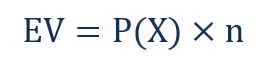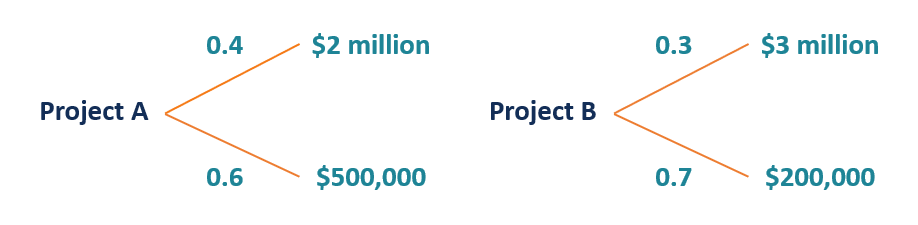# Expected Value

A long-run average value of random variables

## What is Expected Value?

Expected value (also known as EV, expectation, average, mean value) is a long-run average value of random variables. It also indicates the probability-weighted average of all possible values.Expected value is a commonly used financial concept. In finance, it indicates the anticipated value of an investment in the future. By determining the probabilities of possible scenarios, one can determine the EV of the scenarios. The concept is frequently used with multivariate models and scenario analysis. It is directly related to the concept of expected return.

### Formula for Expected Value

The first variation of the expected value formula is the EV of one event repeated several times (think about tossing a coin). In such a case, the EV can be found using the following formula:Where:

• EV – the expected value
• P(X) – the probability of the event
• n – the number of the repetitions of the event

However, in finance, many problems related to the expected value involve multiple events. In such a scenario, the EV is the probability-weighted average of all possible events. Therefore, the general formula to find the EV for multiple events is:Where:

• EV – the expected value
• P(XI) – the probability of the event
• XI – the event

### Example of Expected Value (Multiple Events)

You are the financial analyst in a development company. Your manager just asked you to assess the viability of future development projects and select the most promising one. According to estimates, Project A, upon completion, shows a probability of 0.4 to achieve a value of \$2 million and a probability of 0.6 to achieve a value of \$500,000. Project B shows a probability of 0.3 to be valued at \$3 million and a probability of 0.7 to be valued at \$200,000 upon completion.In order to select the right project, you need to calculate the expected value of each project and compare the values with each other. The EV can be calculated in the following way:

EV (Project A) = [0.4 × \$2,000,000] + [0.6 × \$500,000] = \$1,100,000

EV (Project B) = [0.3 × \$3,000,000] + [0.7 × \$200,000] = \$1,040,000

The EV of Project A is greater than the EV of Project B. Therefore, your company should select Project A.

Note that the example above is an oversimplified one. The real-life example will likely assess the Net Present Value (NPV) of the projects instead of their EV. However, NPV calculations also consider the EV of different projects.

### More Resources

CFI is the official provider of the Financial Modeling and Valuation Analyst (FMVA)™ certification program, designed to transform anyone into a world-class financial analyst.

To keep learning and developing your knowledge of financial analysis, we highly recommend the additional resources below:

• Dependent Variable
• Independent Variable
• Regression Analysis
• Zero Sum (and Non-Zero) Game

### Financial Analyst Certification

Become a certified Financial Modeling and Valuation Analyst (FMVA)® by completing CFI’s online financial modeling classes and training program!